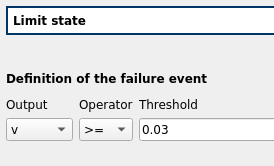Persalys offers the possibility to calculate the reliability of your structures or systems. The goal is to calculate a probability of failure or a probability of threshold exceedance of a quantity of interest. To do so, simply define a limit state specifying if the system fails when it is above or below a critical value.Persalys provides 4 methods:

• Monte Carlo simulation
• FORM or SORM approximation (First/Second Order Reliability Method)
• Importance sampling simulation after the FORM approximation
In addition to the probability of failure, the importance factors or the parameters sensitivity from the distribution to the probability are also provided.

For more information on the use of FORM and SORM methods, refer to the following video:

For more information on the use of the Monte Carlo of Importance sampling simulation, refer to the following video: